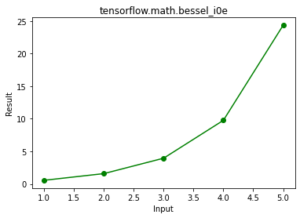Related Articles
Python – tensorflow.math.bessel_i1() function
• Last Updated : 04 Jun, 2020

TensorFlow is open-source Python library designed by Google to develop Machine Learning models and deep learning  neural networks. bessel_i1() is function present in tensorflow math module. This function is used to find element wise first order modified Bessel function.

Syntax: tensorflow.math.bessel_i1( x, name)

Parameters:

• x: It’s a tensor and the allowed dtypes for this tensor are bfloat16, half, float32, float64.
• name: It’s is an optional argument which is used to give operation name.

Returns: It returns a tensor or sparse tensor depending upon x of same dtype as x.

Example 1:

## Python3

 `# importing the library ` `import` `tensorflow as tf ` ` `  `# initializing the input ` `a ``=` `tf.constant([``1``,``2``,``3``,``4``,``5``], dtype ``=` `tf.float64) ` ` `  `# printing the input  ` `print``(``'a: '``,a) ` ` `  `# evaluating the result ` `r ``=` `tf.math.bessel_i1(a) ` ` `  `# printing the result ` `print``(``"Result: "``,r) `

Output:

```a:  tf.Tensor([1. 2. 3. 4. 5.], shape=(5,), dtype=float64)
Result:  tf.Tensor([ 0.5651591   1.59063685  3.95337022  9.75946515 24.33564214], shape=(5,), dtype=float64)

```

Example 2: Visualization

## Python3

 `# importing the library ` `import` `tensorflow as tf ` `import` `matplotlib.pyplot as plt  ` ` `  `# initializing the input ` `a ``=` `tf.constant([``1``,``2``,``3``,``4``,``5``], dtype ``=` `tf.float64) ` ` `  `# evaluating the result ` `r ``=` `tf.math.bessel_i1(a) ` ` `  `#plotting the graph ` `plt.plot(a, r, color ``=` `'green'``, marker ``=` `"o"``)   ` `plt.title(``"tensorflow.math.bessel_i1"``)   ` `plt.xlabel(``"Input"``)   ` `plt.ylabel(``"Result"``)   ` `plt.show()  `

Output:Attention geek! Strengthen your foundations with the Python Programming Foundation Course and learn the basics.

To begin with, your interview preparations Enhance your Data Structures concepts with the Python DS Course.

My Personal Notes arrow_drop_up
Recommended Articles
Page :